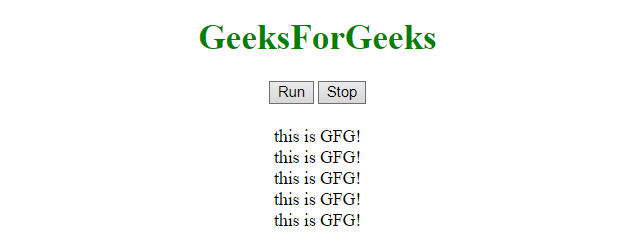# JavaScript | Call a function after a fixed time

In order to run a function multiple times after a fixed amount of time, we are using few functions.
setInterval() Method: This method calls a function at specified intervals(in ms). This method will call continuously the function until clearInterval() is run, or the window is closed.

Syntax:

`setInterval(fun, msec, p1, p2, ...)`

paramters:

• fun: It is required parameter. It holds the function to be executed.
• msec: It is required parameter. The time intervals (in milliseconds) after how long to execute the code. If the value is less than 10, then 10 is used.
• p1, p1, …: It is optional parameter. The parameters pass to the function as an arguments. (Not supported in IE9 and earlier).

clearInterval() Method: This method clears the timer which is set by the setInterval() method. The ID value returned by setInterval() method is used as the parameter for this method.

Syntax:

`clearInterval(varSetInt)`

paramters:

• varSetInt: It is required parameter. This is the name of timer returned by the setInterval() method.

Example 1: This example sets a function, which append a <div> element to the <body> continuously after 2 seconds.

 `  ` `<``html``>  ` `    ``<``head``>  ` `        ``<``title``>  ` `            ``JavaScript | Call a function ` `            ``after a fixed time.  ` `        ``  ` `    ``  ` `     `  `    ``<``body` `style` `= ``"text-align:center;"` `id` `= ``"body"``>  ` `     `  `        ``<``h1` `style` `= ``"color:green;"` `>  ` `            ``GeeksForGeeks  ` `        ``  ` `         `  `        ``<``p` `id` `= ``"GFG_UP"` `style` `= ``"color:green;"``> ` `         `  `        ``<``button` `onclick` `= ``"gfg_Run()"``>  ` `            ``Run ` `        `` ` `         `  `        ``<``p` `id``=``"GFG_DOWN"` `style``=``"color:green;font-size:20px;"``> ` `         `  `        ``<``script``>  ` `            ``var el_up = document.getElementById("GFG_UP"); ` `            ``var el_down = document.getElementById("GFG_DOWN"); ` `            ``var el_body = document.getElementById("body"); ` `            ``el_up.innerHTML = JSON.stringify(GFG_object); ` `             `  `            ``function gfg_Run() {  ` `                ``setInterval(function() { ` `                    ``var node = document.createElement("DIV");          ` `                    ``var textnode = document.createTextNode("this is GFG!"); ` `                    ``node.appendChild(textnode);  ` `                    ``el_body.appendChild(node); ` `                ``}, 2000); ` `            ``} ` `        ``  ` `    ``  ` `                     `

Output:

• Before clicking on the button:• After clicking on the Run button:Example 2: This example sets a function, which appends a <div> element to the <body> continuously after 2 seconds and stops appending when Stop button is clicked.

 `  ` `<``html``>  ` `    ``<``head``>  ` `        ``<``title``>  ` `            ``JavaScript | Call a function after a fixed time.  ` `        ``  ` `    ``  ` `     `  `    ``<``body` `style` `= ``"text-align:center;"` `id` `= ``"body"``>  ` `         `  `        ``<``h1` `style` `= ``"color:green;"` `>  ` `            ``GeeksForGeeks  ` `        ``  ` `         `  `        ``<``p` `id` `= ``"GFG_UP"` `style` `= ``"color:green;"``> ` `         `  `        ``<``button` `onclick` `= ``"gfg_Run()"``>  ` `            ``Run ` `        `` ` `         `  `        ``<``button` `onclick` `= ``"gfg_Stop()"``>  ` `            ``Stop ` `        `` ` `         `  `        ``<``p` `id``=``"GFG_DOWN"` `style``=``"color:green;font-size:20px;"``> ` `         `  `        ``<``script``>  ` `            ``var el_up = document.getElementById("GFG_UP"); ` `            ``var el_down = document.getElementById("GFG_DOWN"); ` `            ``var el_body = document.getElementById("body"); ` `            ``el_up.innerHTML = JSON.stringify(GFG_object); ` `            ``var timer; ` `            ``function gfg_Run() {  ` `                ``timer = setInterval(function() { ` `                    ``var node = document.createElement("DIV");          ` `                    ``var textnode = document.createTextNode("this is GFG!"); ` `                    ``node.appendChild(textnode);  ` `                    ``el_body.appendChild(node); ` `                ``}, 2000); ` `            ``} ` `            ``function gfg_Stop() { ` `                ``clearInterval(timer); ` `             `  `            ``} ` `        ``  ` `    ``  ` `                     `

Output:

• Before clicking on the button:• After clicking on the Run button:Example 3: This example sets a function, which append a <div> element to the <body> continuously after 1 seconds with a different approach than the previous one and stops appending when Stop button is clicked.

 `                        ``  ` `<``html``>  ` `    ``<``head``>  ` `        ``<``title``>  ` `            ``JavaScript | Call a function after a fixed time.  ` `        ``  ` `    ``  ` `     `  `    ``<``body` `style` `= ``"text-align:center;"` `id` `= ``"body"``>  ` `     `  `        ``<``h1` `style` `= ``"color:green;"` `>  ` `            ``GeeksForGeeks  ` `        ``  ` `     `  `        ``<``p` `id` `= ``"GFG_UP"` `style` `= ``"color:green;"``> ` `         `  `        ``<``button` `onclick` `= ``"gfg_Run()"``>  ` `            ``Run ` `        `` ` `         `  `        ``<``button` `onclick` `= ``"gfg_Stop()"``>  ` `            ``Stop ` `        `` ` `         `  `        ``<``p` `id``=``"GFG_DOWN"` `style``=``"color:green;font-size:20px;"``> ` `         `  `        ``<``script``>  ` `            ``var el_up = document.getElementById("GFG_UP"); ` `            ``var el_down = document.getElementById("GFG_DOWN"); ` `            ``var el_body = document.getElementById("body"); ` `            ``el_up.innerHTML = JSON.stringify(GFG_object); ` `            ``var timer; ` `             `  `            ``function gfg_Run() {  ` `                ``function gfg_function() { ` `                    ``var node = document.createElement("DIV");          ` `                    ``var textnode = document.createTextNode("this is GFG!"); ` `                    ``node.appendChild(textnode);  ` `                    ``el_body.appendChild(node); ` `                ``} ` `                ``timer = setInterval(gfg_function, 1000); ` `            ``} ` `            ``function gfg_Stop() { ` `                ``clearInterval(timer); ` `             `  `            ``} ` `        ``  ` `    ``  ` `                     `

Output:

• Before clicking on the button:• After clicking on the Run button:My Personal Notes arrow_drop_upCheck out this Author's contributed articles.

If you like GeeksforGeeks and would like to contribute, you can also write an article using contribute.geeksforgeeks.org or mail your article to contribute@geeksforgeeks.org. See your article appearing on the GeeksforGeeks main page and help other Geeks.

Please Improve this article if you find anything incorrect by clicking on the "Improve Article" button below.